# armax

Estimate parameters of ARMAX, ARIMAX, ARMA, or ARIMA model using time-domain data

## Syntax

``````sys = armax(data,[na nb nc nk])``````
``````sys = armax(data,[na nb nc nk],Name,Value)``````
``sys = armax(data,init_sys)``
``sys = armax(data,___,opt)``
``[sys,ic] = armax(___)``

## Description

### Estimate an ARMAX Model

example

``````sys = armax(data,[na nb nc nk])``` estimates the parameters of an ARMAX or an ARMA `idpoly` model `sys` using the prediction-error method and the polynomial orders specified in `[na nb nc nk]`. The model properties include estimation covariances (parameter uncertainties) and goodness of fit between the estimated and the measured data.```

example

``````sys = armax(data,[na nb nc nk],Name,Value)``` specifies additional options using one or more name-value pair arguments. For instance, using the name-value pair argument `'IntegrateNoise',1` estimates an ARIMAX or ARIMA model, which is useful for systems with nonstationary disturbances.```

### Configure Initial Parameters

example

````sys = armax(data,init_sys)` uses the discrete-time linear model `init_sys` to configure the initial parameterization.```

example

````sys = armax(data,___,opt)` incorporates an option set `opt` that specifies options such as estimation objective, handling of initial conditions, regularization, and numerical search method to use for estimation. Specify `opt` after any of the previous input-argument combinations.```

### Return Estimated Initial Conditions

example

````[sys,ic] = armax(___)` returns the estimated initial conditions as an `initialCondition` object. Use this syntax if you plan to simulate or predict the model response using the same estimation input data and then compare the response with the same estimation output data. Incorporating the initial conditions yields a better match during the first part of the simulation.```

## Examples

collapse all

Estimate an ARMAX model and view the fit of the model output to the estimation data.

Load the measurement data in `iddata` object `z2`.

`load iddata2 z2`

Estimate an ARMAX model with second-order $\mathit{A}$,$\mathit{B}$, and $\mathit{C}$ polynomials and a transport delay of one sample period.

```na = 2; nb = 2; nc = 2; nk = 1; sys = armax(z2,[na nb nc nk])```
```sys = Discrete-time ARMAX model: A(z)y(t) = B(z)u(t) + C(z)e(t) A(z) = 1 - 1.512 z^-1 + 0.7006 z^-2 B(z) = -0.2606 z^-1 + 1.664 z^-2 C(z) = 1 - 1.604 z^-1 + 0.7504 z^-2 Sample time: 0.1 seconds Parameterization: Polynomial orders: na=2 nb=2 nc=2 nk=1 Number of free coefficients: 6 Use "polydata", "getpvec", "getcov" for parameters and their uncertainties. Status: Estimated using ARMAX on time domain data "z2". Fit to estimation data: 85.89% (prediction focus) FPE: 1.086, MSE: 1.054 ```

The output displays the polynomial containing the estimated parameters alongside the estimation details. Under `Status`,` Fit to estimation data` shows that the estimated model has 1-step-ahead prediction accuracy above 80%.

Compare the model simulated output to the measured data.

`compare(z2,sys)`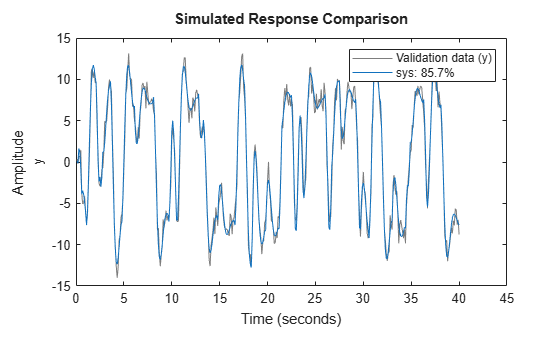The fit of the simulated model to the measured data is nearly the same as the estimation fit.

Estimate an ARMA model and compare its response with both the measured output and an AR model.

Load the data, which contains the time series `z9` with noise.

`load iddata9 z9`

Estimate a fourth-order ARMA model with a first-order $\mathit{C}\text{\hspace{0.17em}}$polynomial.

```na = 4; nc = 1; sys = armax(z9,[na nc]);```

Estimate a fourth-order AR model.

`sys_ar = ar(z9,na);`

Compare the model outputs with the measured data.

`compare(z9,sys,sys_ar)`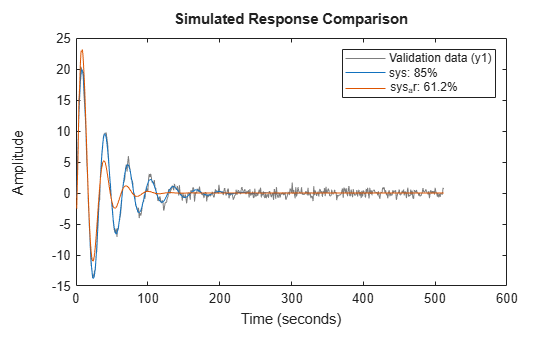The ARMA model has the better fit to the data.

Estimate an ARMAX model from measured data and specify estimation options.

Load the data and create an `iddata` object. Initialize option set `opt`, and set options for `Focus`, `SearchMethod`, `MaxIterations`, and `Display`. Then estimate the ARMAX model using the updated option set.

```load twotankdata; z = iddata(y,u,0.2); opt = armaxOptions; opt.Focus = 'simulation'; opt.SearchMethod = 'lm'; opt.SearchOptions.MaxIterations = 10; opt.Display = 'on'; sys = armax(z,[2 2 2 1],opt);```

The termination conditions for measured component of the model shown in the progress viewer is that the maximum number of iterations were reached.

To improve results, re-estimate the model using a greater value for `MaxIterations`, or continue iterations on the previously estimated model as follows:

```sys2 = armax(z,sys); compare(z,sys,sys2)```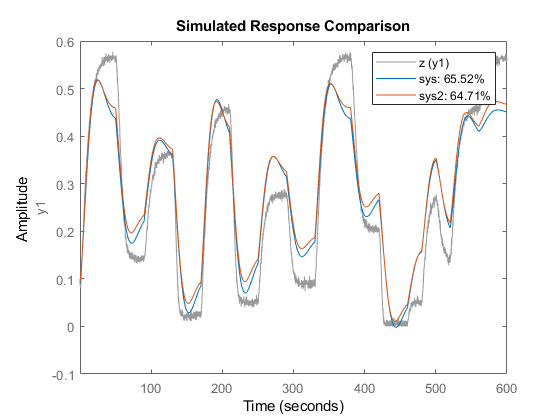where `sys2` refines the parameters of `sys` to improve the fit to data.

Estimate a regularized ARMAX model by converting a regularized ARX model.

`load regularizationExampleData.mat m0simdata;`

Estimate an unregularized ARMAX model of order 30.

`m1 = armax(m0simdata(1:150),[30 30 30 1]);`

Estimate a regularized ARMAX model by determining the Lambda value by trial and error.

```opt = armaxOptions; opt.Regularization.Lambda = 1; m2 = armax(m0simdata(1:150),[30 30 30 1],opt);```

Obtain a lower order ARMAX model by converting a regularized ARX model and then performing order reduction.

```opt1 = arxOptions; [L,R] = arxRegul(m0simdata(1:150),[30 30 1]); opt1.Regularization.Lambda = L; opt1.Regularization.R = R; m0 = arx(m0simdata(1:150),[30 30 1],opt1); mr = idpoly(balred(idss(m0),7));```

Compare the model outputs against the data.

```opt2 = compareOptions('InitialCondition','z'); compare(m0simdata(150:end),m1,m2,mr,opt2);```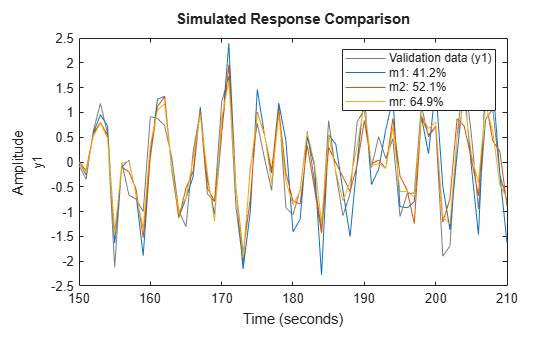Estimate a fourth-order ARIMA model for univariate time-series data.

Load data that contains a time series with noise.

`load iddata9 z9;`

Integrate the output signal and use the result to replace the original output signal in `z9`.

`z9.y = cumsum(z9.y);`

Estimate a fourth-order ARIMA model with a first-order$\mathit{C}$polynomial by setting `'IntegrateNoise`' to `true`.

`model = armax(z9,[4 1],'IntegrateNoise',true); `

Predict the model output using 10-step ahead prediction, and compare the predicted output with the estimation data.

`compare(z9,model,10)`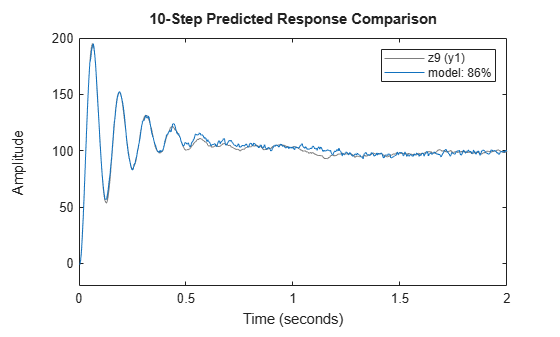Estimate ARMAX models of varying orders iteratively from measured data.

Load `dryer2` data and perform estimation for combinations of polynomial orders `na`, `nb`, `nc`, and input delay `nk`.

```load dryer2; z = iddata(y2,u2,0.08,'Tstart',0); na = 2:4; nc = 1:2; nk = 0:2; models = cell(1,18); ct = 1; for i = 1:3 na_ = na(i); nb_ = na_; for j = 1:2 nc_ = nc(j); for k = 1:3 nk_ = nk(k); models{ct} = armax(z,[na_ nb_ nc_ nk_]); ct = ct+1; end end end```

Stack the estimated models and compare their simulated responses to the estimation data `z`.

```models = stack(1,models{:}); compare(z,models)```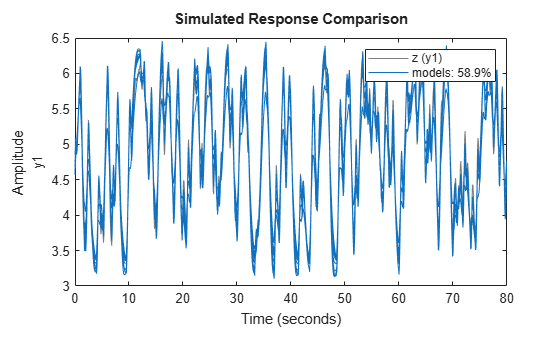`load iddata2 z2`

Estimate a state-space model of order 3 from the estimation data.

`sys0 = n4sid(z2,3);`

Estimate an ARMAX model using the previously estimated state-space model to initialize the parameters.

`sys = armax(z2,sys0);`

`load iddata1ic z1i`

Estimate a second-order ARMAX model `sys` and return the initial conditions in `ic`.

```na = 2; nb = 2; nc = 2; nk = 1; [sys,ic] = armax(z1i,[na nb nc nk]); ic```
```ic = initialCondition with properties: A: [2x2 double] X0: [2x1 double] C: [0 1] Ts: 0.1000 ```

`ic` is an `initialCondition` object that encapsulates the free response of `sys`, in state-space form, to the initial state vector in `X0`. You can incorporate `ic` when you simulate `sys` with the `z1i` input signal and compare the response with the `z1i` output signal.

## Input Arguments

collapse all

Time-domain estimation data, specified as an `iddata` object. For ARMA and ARIMA time-series models, the input channel in `data` must be empty. For examples, see ARMA Model and ARIMA Model.

Polynomial orders and delays for the model, specified as a 1-by-4 vector or vector of matrices `[na nb nc nk]`. The polynomial order is equal to the number of coefficients to estimate in that polynomial.

For an ARMA or ARIMA time-series model, which has no input, set ```[na nb nc nk]``` to `[na nc]`. For an example, see ARMA Model.

For a model with Ny outputs and Nu inputs:

• `na` is the order of the polynomial A(q), specified as an Ny-by-Ny matrix of nonnegative integers.

• `nb` is the order of the polynomial B(q) + 1, specified as an Ny-by-Nu matrix of nonnegative integers.

• `nc` is the order of the polynomial C(q), specified as a column vector of nonnegative integers of length Ny.

• `nk` is the input-output delay, also known at the transport delay, specified as an Ny-by-Nu matrix of nonnegative integers. `nk` is represented in ARMAX models by fixed leading zeros of the B polynomial.

For an example, see Estimate ARMAX Model.

System for configuring the initial parameterization of `sys`, specified as a discrete-time linear model. You obtain `init_sys` by either performing an estimation using measured data or by direct construction using commands such as `idpoly` and `idss`.

If `init_sys` is an ARMAX model, `armax` uses the parameter values of `init_sys` as the initial guess for estimation. To configure initial guesses and constraints for A(q), B(q), and C(q), use the `Structure` property of `init_sys`. For example:

• To specify an initial guess for the A(q) term of `init_sys`, set `init_sys.Structure.A.Value` as the initial guess.

• To specify constraints for the B(q) term of `init_sys`:

• Set `init_sys.Structure.B.Minimum` to the minimum B(q) coefficient values.

• Set `init_sys.Structure.B.Maximum` to the maximum B(q) coefficient values.

• Set `init_sys.Structure.B.Free` to indicate which B(q) coefficients are free for estimation.

If `init_sys` is not a polynomial model with the ARMAX structure, the software first converts `init_sys` to an ARMAX model. `armax` uses the parameters of the resulting model as the initial guess for estimating `sys`.

If `opt` is not specified and `init_sys` was obtained by estimation, then the estimation options from `init_sys.Report.OptionsUsed` are used.

For an example, see Initialize ARMAX Model Parameters Using State-Space Model.

Estimation options for ARMAX model identification, specified as an `armaxOptions` option set. Options specified by `opt` include the following:

• Initial condition handling — Use this option to determine how the initial conditions are set or estimated.

• Input and output data offsets — Use these options to remove offsets from data during estimation.

• Regularization — Use this option to control the tradeoff between bias and variance errors during the estimation process.

### Name-Value Arguments

Specify optional comma-separated pairs of `Name,Value` arguments. `Name` is the argument name and `Value` is the corresponding value. `Name` must appear inside quotes. You can specify several name and value pair arguments in any order as `Name1,Value1,...,NameN,ValueN`.

Example: `'InputDelay',2` applies an input delay of two sample periods to all input channels

Input delays expressed as integer multiples of the sample time, specified as the comma-separated pair consisting of `'InputDelay'` and one of the following:

• Nu-by-1 vector, where Nu is the number of inputs — Each entry is a numerical value representing the input delay for the corresponding input channel.

• Scalar value — Apply the same delay to all input channels.

• `0` — No input delays.

Example: `armax(data,[2 1 1 0],'InputDelay',1)` estimates a second-order ARX model with first-order B and C polynomials that has an input delay of two samples.

Transport delays for each input-output pair, expressed as integer multiples of the sample time, and specified as the comma-separated pair consisting of `'IODelay'` and one of the following:

• Ny-by-Nu matrix, where Ny is the number of outputs and Nu is the number of inputs — Each entry is an integer value representing the transport delay for the corresponding input-output pair.

• Scalar value — Apply the same delay to all input-output pairs.

`'IODelay'` is useful as a replacement for the `nk` order. You can factor out `max(nk-1,0)` lags as the `'IODelay'` value. For `nk` > 1, `armax(na,nb,nk)` is equivalent to `armax(na,nb,1,'IODelay',nk-1)`.

Addition of integrators in the noise channel, specified as the comma-separated pair consisting of `'IntegrateNoise'` and a logical vector of length Ny, where Ny is the number of outputs.

Setting `'IntegrateNoise'` to `true` for a particular output results in the model

`$A\left(q\right)y\left(t\right)=B\left(q\right)u\left(t-nk\right)+\frac{C\left(q\right)}{1-{q}^{-1}}e\left(t\right)$`

where $\frac{1}{1-{q}^{-1}}$ is the integrator in the noise channel, e(t).

Use `'IntegrateNoise'` to create ARIMA or ARIMAX models.

For an example, see ARIMA Model.

## Output Arguments

collapse all

ARMAX model that fits the given estimation data, returned as a discrete-time `idpoly` object. This model is created using the specified model orders, delays, and estimation options.

Information about the estimation results and options used is stored in the `Report` property of the model. `Report` has the following fields.

Report FieldDescription
`Status`

Summary of the model status, which indicates whether the model was created by construction or obtained by estimation.

`Method`

Estimation command used.

`InitialCondition`

Handling of initial conditions during model estimation, returned as one of the following values:

• `'zero'` — The initial conditions were set to zero.

• `'estimate'` — The initial conditions were treated as independent estimation parameters.

• `'backcast'` — The initial conditions were estimated using the best least squares fit.

This field is especially useful to view how the initial conditions were handled when the `InitialCondition` option in the estimation option set is `'auto'`.

`Fit`

Quantitative assessment of the estimation, returned as a structure. See Loss Function and Model Quality Metrics for more information on these quality metrics. The structure has the following fields:

FieldDescription
`FitPercent`

Normalized root mean squared error (NRMSE) measure of how well the response of the model fits the estimation data, expressed as the percentage fitpercent = 100(1-NRMSE).

`LossFcn`

Value of the loss function when the estimation completes.

`MSE`

Mean squared error (MSE) measure of how well the response of the model fits the estimation data.

`FPE`

Final prediction error for the model.

`AIC`

Raw Akaike Information Criteria (AIC) measure of model quality.

`AICc`

Small-sample-size corrected AIC.

`nAIC`

Normalized AIC.

`BIC`

Bayesian Information Criteria (BIC).

`Parameters`

Estimated values of model parameters.

`OptionsUsed`

Option set used for estimation. If no custom options were configured, this is a set of default options. See `armaxOptions` for more information.

`RandState`

State of the random number stream at the start of estimation. Empty, `[]`, if randomization was not used during estimation. For more information, see `rng`.

`DataUsed`

Attributes of the data used for estimation, returned as a structure with the following fields.

FieldDescription
`Name`

Name of the data set.

`Type`

Data type.

`Length`

Number of data samples.

`Ts`

Sample time.

`InterSample`

Input intersample behavior, returned as one of the following values:

• `'zoh'` — Zero-order hold maintains a piecewise-constant input signal between samples.

• `'foh'` — First-order hold maintains a piecewise-linear input signal between samples.

• `'bl'` — Band-limited behavior specifies that the continuous-time input signal has zero power above the Nyquist frequency.

`InputOffset`

Offset removed from time-domain input data during estimation. For nonlinear models, it is `[]`.

`OutputOffset`

Offset removed from time-domain output data during estimation. For nonlinear models, it is `[]`.

`Termination`

Termination conditions for the iterative search used for prediction error minimization, returned as a structure with the following fields:

FieldDescription
`WhyStop`

Reason for terminating the numerical search.

`Iterations`

Number of search iterations performed by the estimation algorithm.

`FirstOrderOptimality`

$\infty$-norm of the gradient search vector when the search algorithm terminates.

`FcnCount`

Number of times the objective function was called.

`UpdateNorm`

Norm of the gradient search vector in the last iteration. Omitted when the search method is `'lsqnonlin'` or `'fmincon'`.

`LastImprovement`

Criterion improvement in the last iteration, expressed as a percentage. Omitted when the search method is `'lsqnonlin'` or `'fmincon'`.

`Algorithm`

Algorithm used by `'lsqnonlin'` or `'fmincon'` search method. Omitted when other search methods are used.

For estimation methods that do not require numerical search optimization, the `Termination` field is omitted.

For more information on using `Report`, see Estimation Report.

Estimated initial conditions, returned as an `initialCondition` object or an object array of `initialCondition` values.

• For a single-experiment data set, `ic` represents, in state-space form, the free response of the transfer function model (A and C matrices) to the estimated initial states (x0).

• For a multiple-experiment data set with Ne experiments, `ic` is an object array of length Ne that contains one set of `initialCondition` values for each experiment.

If `armax` returns `ic` values of `0` and the you know that you have non-zero initial conditions, set the `'InitialCondition'` option in `armaxOptions` to `'estimate'` and pass the updated option set to `armax`. For example:

```opt = armaxOptions('InitialCondition','estimate') [sys,ic] = armax(data,np,nz,opt)```
The default `'auto'` setting of `'InitialCondition'` uses the `'zero'` method when the initial conditions have a negligible effect on the overall estimation-error minimization process. Specifying `'estimate'` ensures that the software estimates values for `ic`.

For more information, see `initialCondition`. For an example of using this argument, see Obtain Initial Conditions.

collapse all

### ARMAX Model

The ARMAX (Autoregressive Moving Average with Extra Input) model structure is:

A more compact way to write the difference equation is

`$A\left(q\right)y\left(t\right)=B\left(q\right)u\left(t-{n}_{k}\right)+C\left(q\right)e\left(t\right)$`

where

• $y\left(t\right)$ — Output at time $t$

• ${n}_{a}$ — Number of poles

• ${n}_{b}$ — Number of zeroes plus 1

• ${n}_{c}$ — Number of C coefficients

• ${n}_{k}$ — Number of input samples that occur before the input affects the output, also called the dead time in the system

• $y\left(t-1\right)\dots y\left(t-{n}_{a}\right)$ — Previous outputs on which the current output depends

• $u\left(t-{n}_{k}\right)\dots u\left(t-{n}_{k}-{n}_{b}+1\right)$ — Previous and delayed inputs on which the current output depends

• $e\left(t-1\right)\dots e\left(t-{n}_{c}\right)$ — White-noise disturbance value

The parameters `na`, `nb`, and `nc` are the orders of the ARMAX model, and `nk` is the delay. q is the delay operator. Specifically,

`$A\left(q\right)=1+{a}_{1}{q}^{-1}+\dots +{a}_{{n}_{a}}{q}^{-{n}_{a}}$`
`$B\left(q\right)={b}_{1}+{b}_{2}{q}^{-1}+\dots +{b}_{{n}_{b}}{q}^{-{n}_{b}+1}$`
`$C\left(q\right)=1+{c}_{1}{q}^{-1}+\dots +{c}_{{n}_{c}}{q}^{-{n}_{c}}$`

### ARMA Time-Series Model

The ARMA (Autoregressive Moving Average) model is a special case of an ARMAX Model with no input channels. The ARMA single-output model structure is given by the following equation:

`$A\left(q\right)y\left(t\right)=C\left(q\right)e\left(t\right)$`

### ARIMAX Model

The ARIMAX (Autoregressive Integrated Moving Average with Extra Input) model structure is similar to the ARMAX model, except that it contains an integrator in the noise source e(t):

`$A\left(q\right)y\left(t\right)=B\left(q\right)u\left(t-nk\right)+\frac{C\left(q\right)}{\left(1-{q}^{-1}\right)}e\left(t\right)$`

### ARIMA Model

The ARIMA (Autoregressive Integrated Moving Average) model structure is a reduction of the ARIMAX model with no inputs:

`$A\left(q\right)y\left(t\right)=\frac{C\left(q\right)}{\left(1-{q}^{-1}\right)}e\left(t\right)$`

## Algorithms

An iterative search algorithm minimizes a robustified quadratic prediction error criterion. The iterations are terminated when any of the following is true:

• Maximum number of iterations is reached.

• Expected improvement is less than the specified tolerance.

• Lower value of the criterion cannot be found.

You can get information about the stopping criteria using `sys.Report.Termination`.

Use the `armaxOptions` option set to create and configure options affecting the estimation results. In particular, set the search algorithm attributes, such as `MaxIterations` and `Tolerance`, using the `'SearchOptions'` property.

When you do not specify initial parameter values for the iterative search as an initial model, they are constructed in a special four-stage LS-IV algorithm.

The cutoff value for the robustification is based on the `Advanced.ErrorThreshold` estimation option and on the estimated standard deviation of the residuals from the initial parameter estimate. The cutoff value is not recalculated during the minimization. By default, no robustification is performed; the default value of `ErrorThreshold` option is 0.

To ensure that only models corresponding to stable predictors are tested, the algorithm performs a stability test of the predictor. Generally, both $C\left(q\right)$ and $F\left(q\right)$ (if applicable) must have all zeros inside the unit circle.

Minimization information is displayed on the screen when the estimation option `'Display'` is `'On'` or `'Full'`. When `'Display'` is `'Full'`, both the current and the previous parameter estimates are displayed in column-vector form, and the parameters are listed in alphabetical order. Also, the values of the criterion function (cost) are given and the Gauss-Newton vector and its norm are displayed. When `'Display'` is `'On'`, only the criterion values are displayed.

## Alternatives

`armax` does not support continuous-time model estimation. Use `tfest` to estimate a continuous-time transfer function model, or `ssest` to estimate a continuous-time state-space model.

`armax` supports only time-domain data. For frequency-domain data, use `oe` to estimate an Output-Error (OE) model.

 Ljung, L. System Identification: Theory for the User, Second Edition. Upper Saddle River, NJ: Prentice-Hall PTR, 1999. See chapter about computing the estimate.

## SupportGet trial now